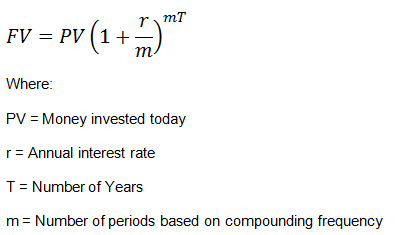# Future Value of a Single Cash Flow

Future value of a single cash flow refers to how much a single cash flow today would grow to over a period of time if put in an investment that pays compound interest.

The formula for calculating future value is:Example

Calculate the future value (FV) of an investment of $500 for a period of 3 years that pays an interest rate of 6% compounded semi-annually. FV = 500*(1+6%/2)^(2*3) =$597.03

We can also solve this problem using the calculator as follows:

Calculator Variables

The BA II Plus calculator has the following five variables for Time Value of Money (TVM) functions.

N = Number of Periods (mT in our formula)

I/Y = Interest Rate Per Year (r)

PV = Present Value

FV = Future Value

PMT = Payment

The calculations are simple; you input the values that you know, and calculate the unknown.

To assign a value to a TVM variable, key in the number and press a TVM key – N, I/Y, PV, PMT, and FV.

To change the number of payments (P/Y) press 2nd, key in the number and press ENTER.

To calculate the unknown value, press Compute (CPT) and then press the key for unknown variable.

In our above example, enter PV = 500, change P/Y = 2 (semi-annual compounding), I/Y = 6, N = 6.

Then press CPT > FV. We get:

FV = 597.026

Similarly we can calculate the Future Value for any compounding frequency.